TitleIntermediate Algebra
Answer/Discussion to Practice Problems
Tutorial 15: The Slope of a LineAnswer/Discussion to 1a (3, 5) and (-1, -8)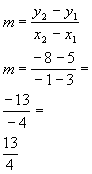*Plug in x and y values into slope formula *Simplify

 The slope would be 13/4. (return to problem 1a)Answer/Discussion to 1b (4, 2) and (4, -2)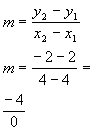*Plug in x and y values into slope formula *Simplify

 The slope would be undefined. (return to problem 1b)Answer/Discussion to 2a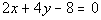First, we need to write it in the slope/intercept form: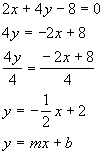*Sub. 2x from both sides *Inverse of mult. by 4 is div. by 4 *Written in slope/intercept form

 Lining up the form with the equation we got, can you see what the slope and y-intercept are? It looks like our slope is -1/2 and our y-intercept is 2. (return to problem 2a)Answer/Discussion to 2b x = -2 Note how we do not have a y.  This type of linear equation was shown in Tutorial 14 (Graphing Linear Equations).  When we have x = c, where c is a constant, then this graph is what type of line? If you said vertical, you are correct.  Since this is a special type of linear equation that can't be written in the slope/intercept form, I'm going to give you a visual of what is happening and then from that let's see if we can't figure out the slope and y-intercept. The graph would look like this: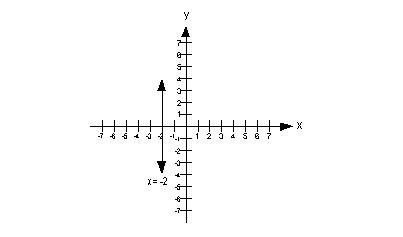First, let's talk about the slope.  Note that all the x values on this graph are -2.  That means the change in x, which is the denominator of the slope formula, would be -2 - (-2) = 0.  Well you know that having a 0 in the denominator is a big no no.  This means the slope is undefined.  As shown above, whenever you have a vertical line your slope is undefined. Now, let's look at the y-intercept.  Looking at the graph, you can see that this graph never crosses the y-axis, therefore there is no y-intercept either.  Another way to look at this is the x value has to be 0 when looking for the y-intercept and in this problem x is always -2. Final answer, the slope is undefined and the y-intercept does not exist. (return to problem 2b)Answer/Discussion to 2c y = -1 Note how we do not have an x.  This type of linear equation was shown in Tutorial 14 (Graphing Linear Equations).  When we have y = c, where c is a constant, then this graph is what type of line? If you said horizontal, you are correct.  Since this is a special type of linear equation that can't be written in the slope/intercept form, I'm going to give you a visual of what is happening and then from that let's see if we can't figure out the slope and y-intercept.   The graph would look like this: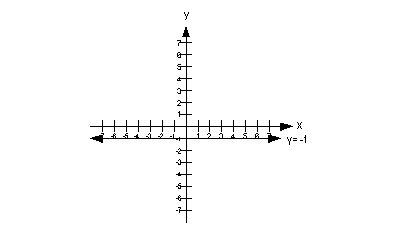First, let's talk about the slope.  Note how all of the y values on this graph are -1.  That means the change in y, which is the numerator of the slope formula would be -1 - (-1) = 0. Having 0 in the numerator and a non-zero number in the denominator means only one thing.  The slope equals 0. Now, let's look at the y-intercept.  Looking at the graph, you can see that this graph crosses the y-axis at (0, -1).  So the y-intercept is (0, -1). The slope is 0 and the y-intercept is -1. (return to problem 2c)Answer/Discussion to 3aand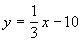Rewriting the first equation in slope/intercept form we get: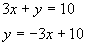*Sub. 3x from both sides

 The slope of that first equation is -3 and the slope of the 2nd equation is 1/3.  It appears that these slopes are negative reciprocals of each other, so that means the lines would have to be perpendicular to each other.  (return to problem 3a)Answer/Discussion to 3b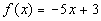and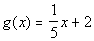The equations are already in the slope/intercept form, so let's go right to looking for the slope.  What did you find?  I found that the slope of the first equation is -5 and the slope of the second equation is 1/5.  So what does that mean?  Well, since the two slopes are negative reciprocals of each other, the lines have to be perpendicular. (return to problem 3b)Answer/Discussion to 4a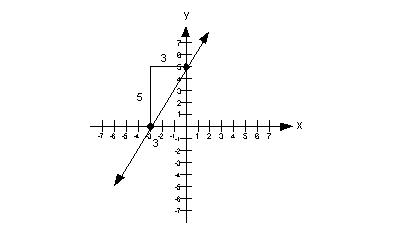The slope = rise/run = 5/3. (return to problem 4a)

Last revised on July 3, 2011 by Kim Seward.
All contents copyright (C) 2001 - 2011, WTAMU and Kim Seward. All rights reserved.